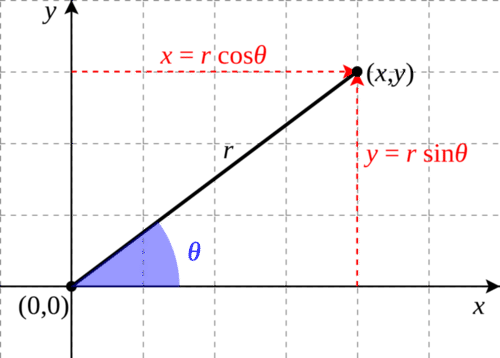# 12.3: Polar Coordinates

•• Contributed by CK12
• CK12

Cartesian coordinates ($$x,y$$) are not the only way of labeling a point $$P$$ on a flat plane by a pair of numbers. Other ways exist, and they can be more useful in special situations.

One system (“polar coordinates") uses the length $$r$$ of the line $$OP$$ from the origin to $$P$$ (i. e. the distance of $$P$$ distance to the origin) and the angle that line makes with the x-axis. Angles are often denoted by Greek letters, and here we follow conventions by marking it with $$\theta$$. Note that while in the cartesian system $$x$$ and $$y$$ play very similar roles, here roles are divided: $$r$$ gives distance and $$\theta$$ direction.

The two representations are closely related. From the definitions of the sine and cosine:

$x\,=\,r\,\times\, \cos \theta$

$y\,=\,r\,\times\, \sin \theta$

This allows $$(x,y$$) to be derived from polar coordinates. This relationship is illustrated below:Figure $$\PageIndex{1}$$: Converting from polar to cartesian coordinates.

To go in the reverse direction, we can use the Pythagorean theorem to find $$r$$:

$r^2\,=\,x^2\,+\,y^2$

Once $$r$$ is known, the rest is easy:

$\cos \theta\,=\frac{x}{r}$

$\sin \theta\,=\frac{y}{r}$

These relations fail only at the origin, where $$x\,=\,y\,=\,r\,=\,0$$. At that point, $$\theta$$ is undefined and one can choose for it whatever one pleases.

In three dimensional space, the cartesian labeling ($$x,y,z$$) is nicely symmetric, but sometimes it is convenient to follow the style of polar coordinates and label distance and direction separately. Distance is easy: you take the line OP from the origin to the point and measure its length r. You can even show from the theorem of Pythagoras that in this case

$r^2\,=\,x^2\,+\,y^2\,+\,z^2$

All the points with the same value of $$r$$ form a sphere of radius $$r$$ around the origin $$O$$. On a sphere we can label each point by latitude $$\lambda$$ (lambda, small Greek L) and longitude $$\phi$$ (phi, small Greek F), so that the position of any point in space is defined by the 3 numbers ($$r,\lambda ,\phi$$).